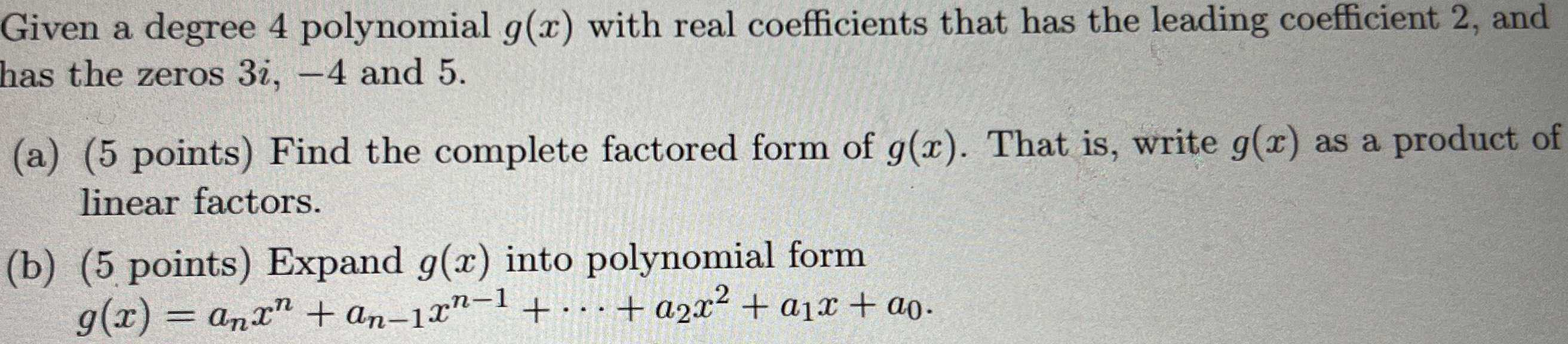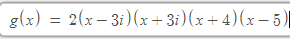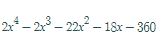### Still have math questions?

Algebra
QuestionGiven a degree $$4$$ polynomial $$g ( x )$$ with real coefficients that has the leading coefficient $$2$$ , and has the zeros $$3 i , - 4$$ and $$5$$ .

(a) ( $$5$$ points) Find the complete factored form of $$g ( x )$$ . That is, write $$g ( x )$$ as a product of linear factors.

(b) $$( 5$$ points) Expand $$g ( x )$$ into polynomial form

$$g ( x ) = a _ { n } x ^ { n } + a _ { n - 1 } x ^ { n - 1 } + \cdots + a _ { 2 } x ^ { 2 } + a _ { 1 } x + a _ { 0 }$$ .